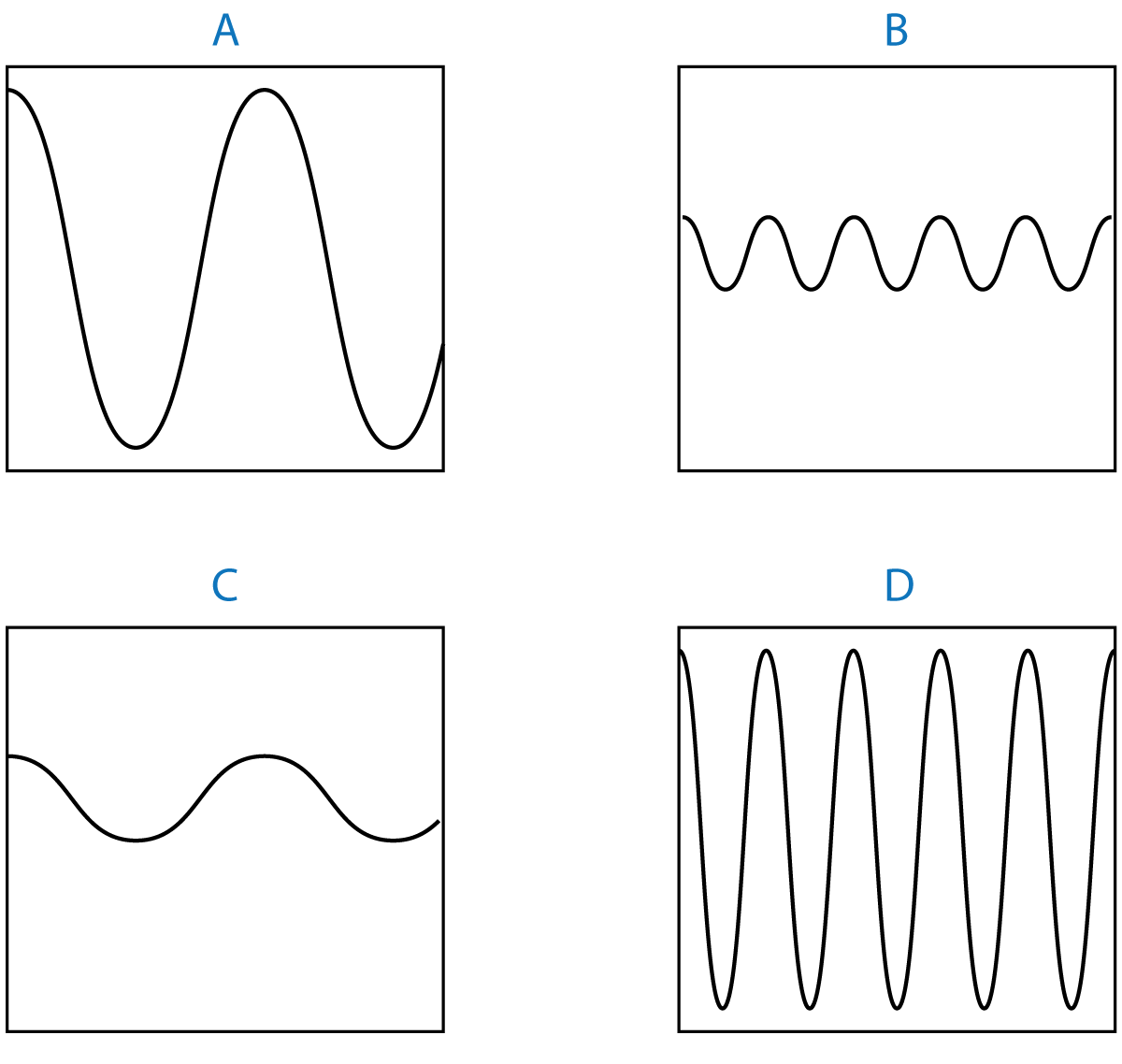10 minutes maximum! Can you do it in 5? Q 1&2. This diagram shows a wave at sea.Which arrow correctly shows ...... 1. ...the wavelength? ABCD 2. ....the amplitude? ABCD 3. A sound wave is produced by a loudspeaker. If the volume is increased, the sound wave transfers more energy. Which of the following measurements has changed to increase the volume? A. The frequency. B. The amplitude. C. The wavelength. D. The wave speed. ABCD An oscilloscope is a device that can be used to display waves. 4 sound waves are shown here:Which of these waves .... 4. ..shows a high amplitude, long wavelength sound? ABCD 5. ..is a low amplitude, high frequency sound? ABCD 6. What is the name given to waves where the oscillations are in the same direction as the wave energy? ? Longitudinal Unidirectional Transverse Polarised Refracted 7. What is the name given to waves where the oscillations are at right angles to the wave energy? ? Longitudinal Unidirectional Transverse Polarised Refracted 8. Which one of these is a longitudinal wave? A. Sound waves. B. Water waves (on the surface). C. Light waves. D. Microwaves. ABCD 9. The number of waves passing a point each second is the... A. period. B. frequency. C. wavelength. D. wave speed. ABCD 10. What is the unit used to measure the number of waves per second? A. watts (W) B. meters per second (m/s) C. decibels (dB) D. hertz (Hz) ABCD